# Subgroup structure of direct product of D8 and Z2

This article gives specific information, namely, subgroup structure, about a particular group, namely: direct product of D8 and Z2.
View subgroup structure of particular groups | View other specific information about direct product of D8 and Z2

This article discusses the subgroup structure of the direct product of D8 and Z2.

A presentation for the group that we use is:$G := \langle a,x,y \mid a^4 = x^2 = y^2 = e, xax = a^{-1}, ya = ay, xy = yx \rangle$.

The group has the following subgroups:

1. The trivial group. (1)
2. The cyclic group$\langle a^2 \rangle$ of order two. This equals the commutator subgroup, is central, and is also the set of squares. Isomorphic to cyclic group:Z2. (1)
3. The subgroups$\langle y \rangle$ and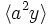$\langle a^2y \rangle$. These are both central subgroups of order two, but are related by an outer automorphism. Isomorphic to cyclic group:Z2. (2)
4. The subgroups$\langle x \rangle$,$\langle ax \rangle$,$\langle a^2x \rangle$,$\langle a^3x \rangle$,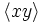$\langle xy \rangle$,$\langle axy \rangle$,$\langle a^2xy$, and$\langle a^3xy \rangle$. These are all related by automorphisms, and are all 2-subnormal subgroups. They come in four conjugacy classes, namely the class$\langle x \rangle, \langle a^2x \rangle$, the class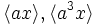$\langle ax \rangle, \langle a^3x \rangle$, the class$\langle xy \rangle, \langle a^2xy$, and the class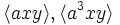$\langle axy \rangle, \langle a^3xy \rangle$. Isomorphic to cyclic group:Z2. (8)
5. The subgroup$\langle a^2, y \rangle$. This is the center, hence is a characteristic subgroup. Isomorphic to Klein four-group. (1)
6. The subgroups$\langle a^2, x \rangle$,$\langle a^2, ax \rangle$,$\langle a^2, xy \rangle$, and$\langle a^2, axy \rangle$. These are all normal subgroups, but are related by outer automorphisms. Isomorphic to Klein four-group. (4)
7. The subgroups$\langle y, x \rangle$,$\langle y, ax \rangle$,$\langle y, a^2x \rangle$,$\langle y, a^3x$,$\langle a^2y, x \rangle$,$\langle a^2y, ax$,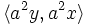$\langle a^2y, a^2x \rangle$,$\langle a^2y, a^3x \rangle$. These subgroups are all 2-subnormal subgroups and are related by outer automorphisms, and they come in four conjugacy classes of size two. Isomorphic to Klein four-group. (8)
8. The subgroups$\langle a \rangle$ and$\langle ay \rangle$. They are both normal and are related via an outer automorphism. Isomorphic to cyclic group:Z4. (2)
9. The subgroups$\langle a^2,x,y \rangle$ and$\langle a^2, ax, y \rangle$. These are normal and are related by outer automorphisms. Isomorphic to elementary abelian group of order eight. (2)
10. The subgroups$\langle a,x \rangle$,$\langle a, xy \rangle$,$\langle ay, x \rangle$ and$\langle ay, xy \rangle$. These are all normal and are related by an outer automorphism. Isomorphic to dihedral group:D8. (4)
11. The subgroup$\langle a, y \rangle$. This is a characteristic subgroup. Isomorphic to direct product of Z4 and Z2. (1)
12. The whole group. (1)

## Tables for quick information

### Table classifying isomorphism types of subgroups

Group name GAP ID Occurrences as subgroup Conjugacy classes of occurrence as subgroup Occurrences as normal subgroup Occurrences as characteristic subgroup
Trivial group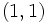$(1,1)$ 1 1 1 1
Cyclic group:Z2$(2,1)$ 11 7 3 1
Cyclic group:Z4$(4,1)$ 2 2 2 0
Klein four-group$(4,2)$ 13 9 5 1
Direct product of Z4 and Z2$(8,2)$ 1 1 1 1
Dihedral group:D8$(8,3)$ 4 4 4 0
Elementary abelian group of order eight$(8,5)$ 2 2 2 0
Direct product of D8 and Z2$(16,11)$ 1 1 1 1
Total -- 35 27 19 5

### Table listing number of subgroups by order

Group name Occurrences as subgroup Conjugacy classes of occurrence as subgroup Occurrences as normal subgroup Occurrences as characteristic subgroup$1$ 1 1 1 1$2$ 11 7 3 1$4$ 15 11 7 1$8$ 7 7 7 1$16$ 1 1 1 1
Total 35 27 19 5

## The commutator subgroup (type (2))

This is the two-element subgroup generated by$a^2$.

### Subgroup properties satisfied by this subgroup

On account of being a commutator subgroup as well as an agemo subgroup, this subgroup is a verbal subgroup. Thus, it satisfies the following subgroup properties:

It also satisfies the following properties:

## The center (type (5))

This is a Klein four-subgroup comprising the identity,$a^2$,$y$ and$a^2y$.

## The unique characteristic subgroup of order eight (type (10))

This is a subgroup generated by$a$ and$y$, and is the direct product of a cyclic group of order four generated by$a$ and a cyclic group of order two generated by$y$.